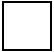Skill 3E
Simple Algebra: Subtraction

Here, we are teaching how to use the opposite operation and fact families to solve a horizontal subtraction problem. This is really the same as "counting-up" to find the answer and the preferred method for this type of problem.

 12 ─ 7 =7 += 12

 15 ─ 9 =9 += 15